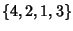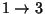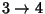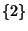## Cycle (Permutation)

A Subset of a Permutation whose elements trade places with one another. A cycle decomposition of a Permutation can therefore be viewed as a Class of a Permutation Group. For example, in the Permutation Group,is a 3-cycle (,, and) andis a 1-cycle (). Every Permutation Group onsymbols can be uniquely expressed as a product of disjoint cycles. The cyclic decomposition of a Permutation can be computed in Mathematica(Wolfram Research, Champaign, IL) with the function ToCycles and the Permutation corresponding to a cyclic decomposition can be computed with FromCycles. According to Vardi (1991), the Mathematica code for ToCycles is one of the most obscure ever written.

To find the numberofcycles in a Permutation Group of order, takewhereis the Stirling Number of the First Kind.